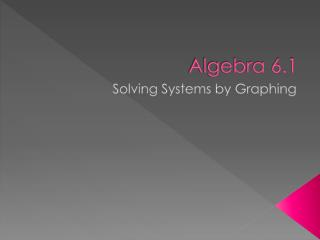Download PresentationAlgebra 6.1

# Algebra 6.1 - PowerPoint PPT Presentation

Algebra 6.1. Solving Systems by Graphing. Learning Targets. Language Goal Students will be able to identify solutions of systems of linear equations in two variables. Math Goal Students will be able to solve systems of linear equations in two variables by graphing. Essential QuestionsI am the owner, or an agent authorized to act on behalf of the owner, of the copyrighted work described.
Download Presentation## Algebra 6.1

An Image/Link below is provided (as is) to download presentation

Download Policy: Content on the Website is provided to you AS IS for your information and personal use and may not be sold / licensed / shared on other websites without getting consent from its author.While downloading, if for some reason you are not able to download a presentation, the publisher may have deleted the file from their server.

- - - - - - - - - - - - - - - - - - - - - - - - - - E N D - - - - - - - - - - - - - - - - - - - - - - - - - -
Presentation Transcript
1. Algebra 6.1 Solving Systems by Graphing

2. Learning Targets Language Goal • Students will be able to identify solutions of systems of linear equations in two variables. Math Goal • Students will be able to solve systems of linear equations in two variables by graphing. Essential Questions • When do we see systems of equations around us today?

3. Warm-up

4. Go over Chapter 5 Test

5. Vocabulary • System of linear equations: • Solution of a system of linear equations: A set of two or more linear equations containing two or more variables An ordered pair that satisfies each equation in the system

6. What is a system? • Two linear equations with 2 variables • Example: • When you find the solution you are finding the point of intersection. • Link to demonstration. • http://my.hrw.com/math06_07/nsmedia/tools/Graph_Calculator/graphCalc.html

7. Example 3: Word Applications A. Bowl-o-rama charges \$2.50 per game plus \$2 for shoe rental, and Bowling Pinz charges \$2 per game plus \$4 for shoe rental. For how many games will the cost to bowl be the same at both places? What is that cost?

8. Example 3: Word Applications B. Wren and Jenni are reading the same book. Wren is on page 14 and reads 2 pages every night. Jenni is on page 6 and reads 3 pages every night. After how many nights will they have read the same number of pages? How many pages will that be?

9. 6.1 Worksheet Directions • Start solving both equations for y. • Graph both lines. • Find the intersection. • Use the website to check your graphs and intersections. • http://my.hrw.com/math06_07/nsmedia/tools/Graph_Calculator/graphCalc.html

10. 6.1 Worksheet • 1.

11. 6.1 Worksheet • 2.

12. 6.1 Worksheet • 3.

13. 6.1 Worksheet • 4.

14. 6.1 Worksheet • 5.

15. 6.1 Worksheet • 6.

16. 6.1 Worksheet • 7.

17. 6.1 Worksheet • 8.

18. 6.1 Worksheet • 9.

19. 6.1 Worksheet • 10.

20. 6.1 Worksheet • 11.

21. 6.1 Worksheet • 12.

22. Lesson Quiz